# Tutorial for Hatch¶

## Create hatches with one boundary path¶

The simplest form of the `Hatch` entity has one polyline path with only straight lines as boundary path:

```import ezdxf

doc = ezdxf.new('R2000')  # hatch requires the DXF R2000 (AC1015) format or later
msp = doc.modelspace()  # adding entities to the model space

hatch = msp.add_hatch(color=2)  # by default a solid fill hatch with fill color=7 (white/black)

# every boundary path is always a 2D element
# vertex format for the polyline path is: (x, y[, bulge])
# there are no bulge values in this example
hatch.paths.add_polyline_path([(0, 0), (10, 0), (10, 10), (0, 10)], is_closed=True)

doc.saveas("solid_hatch_polyline_path.dxf")
```

But like all polyline entities the polyline path can also have bulge values:

```import ezdxf

doc = ezdxf.new('R2000')  # hatch requires the DXF R2000 (AC1015) format or later
msp = doc.modelspace()  # adding entities to the model space

hatch = msp.add_hatch(color=2)  # by default a solid fill hatch with fill color=7 (white/black)

# every boundary path is always a 2D element
# vertex format for the polyline path is: (x, y[, bulge])
# bulge value 1 = an arc with diameter=10 (= distance to next vertex * bulge value)
# bulge value > 0 ... arc is right of line
# bulge value < 0 ... arc is left of line
hatch.paths.add_polyline_path([(0, 0, 1), (10, 0), (10, 10, -0.5), (0, 10)], is_closed=True)

doc.saveas("solid_hatch_polyline_path_with_bulge.dxf")
```

The most flexible way to define a boundary path is the edge path. An edge path consist of a number of edges and each edge can be one of the following elements:

• line `EdgePath.add_line()`
• arc `EdgePath.add_arc()`
• ellipse `EdgePath.add_ellipse()`
• spline `EdgePath.add_spline()`

Create a solid hatch with an edge path (ellipse) as boundary path:

```import ezdxf

doc = ezdxf.new('R2000')  # hatch requires the DXF R2000 (AC1015) format or later
msp = doc.modelspace()  # adding entities to the model space

# important: major axis >= minor axis (ratio <= 1.)
# minor axis length = major axis length * ratio
msp.add_ellipse((0, 0), major_axis=(0, 10), ratio=0.5)

# by default a solid fill hatch with fill color=7 (white/black)
hatch = msp.add_hatch(color=2)

# every boundary path is always a 2D element
edge_path = hatch.paths.add_edge_path()
# each edge path can contain line arc, ellipse and spline elements
# important: major axis >= minor axis (ratio <= 1.)
edge_path.add_ellipse((0, 0), major_axis=(0, 10), ratio=0.5)

doc.saveas("solid_hatch_ellipse.dxf")
```

## Create hatches with multiple boundary paths (islands)¶

The DXF atribute `hatch_style` defines the island detection style:

 0 nested - altering filled and unfilled areas 1 outer - area between external and outermost path is filled 2 ignore - external path is filled
```hatch = msp.add_hatch(color=1, dxfattribs={
'hatch_style': 0,
# 0 = nested
# 1 = outer
# 2 = ignore
})

# The first path has to set flag: 1 = external
# flag const.BOUNDARY_PATH_POLYLINE is added (OR) automatically
hatch.paths.add_polyline_path([(0, 0), (10, 0), (10, 10), (0, 10)], is_closed=True, flags=1)
```

This is also the result for all 4 paths and `hatch_style` set to `2` (ignore).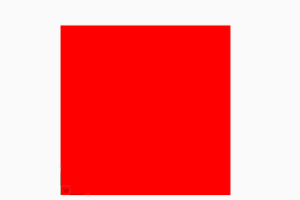```# The second path has to set flag: 16 = outermost
hatch.paths.add_polyline_path([(1, 1), (9, 1), (9, 9), (1, 9)], is_closed=True, flags=16)
```

This is also the result for all 4 paths and `hatch_style` set to `1` (outer).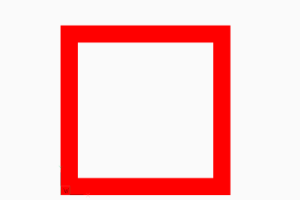```# The third path has to set flag: 0 = default
hatch.paths.add_polyline_path([(2, 2), (8, 2), (8, 8), (2, 8)], is_closed=True, flags=0)
```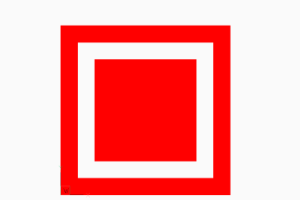```# The forth path has to set flag: 0 = default, and so on
hatch.paths.add_polyline_path([(3, 3), (7, 3), (7, 7), (3, 7)], is_closed=True, flags=0)
```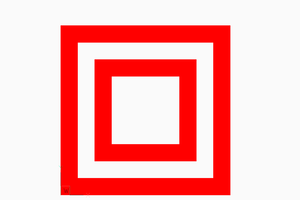The expected result of combinations of various `hatch_style` values and paths flags, or the handling of overlapping paths is not documented by the DXF reference, so don’t ask me, ask Autodesk or just try it by yourself and post your experience in the forum.

## Example for Edge Path Boundary¶

```hatch = msp.add_hatch(color=1)

# 1. polyline path
hatch.paths.add_polyline_path([
(240, 210, 0),
(0, 210, 0),
(0, 0, 0.),
(240, 0, 0),
],
is_closed=1,
flags=1,
)
# 2. edge path
edge_path = hatch.paths.add_edge_path(flags=16)
edge_path.add_spline(
control_points=[
(126.658105895725, 177.0823706957212),
(141.5497003747484, 187.8907860433995),
(205.8997365206943, 154.7946313459515),
(113.0168862297068, 117.8189380884978),
(202.9816918983783, 63.17222935389572),
(157.363511042264, 26.4621294342132),
(144.8204003260554, 28.4383294369643)
],
knot_values=[
0.0, 0.0, 0.0, 0.0, 55.20174685732758, 98.33239645153571,
175.1126541251052, 213.2061566683142, 213.2061566683142,
213.2061566683142, 213.2061566683142
],
)
edge_path.add_arc(
center=(152.6378550678883, 128.3209356351659),
radius=100.1880612627354,
start_angle=94.4752130054052,
end_angle=177.1345242028005,
)
edge_path.add_line(
(52.57506282464041, 123.3124200796114),
(126.658105895725, 177.0823706957212)
)
```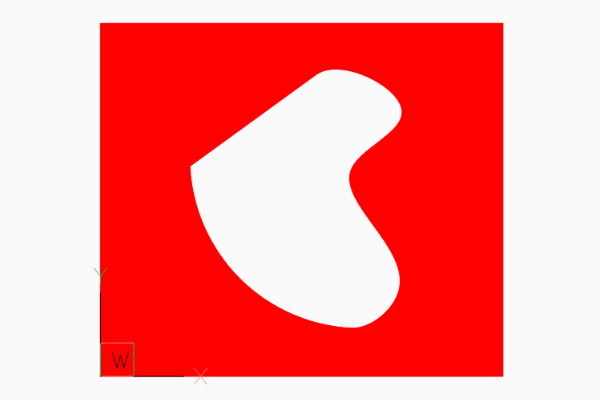## Associative Boundary Paths¶

A HATCH entity can be associative to a base geometry, which means if the base geometry is edited in a CAD application the HATCH get the same modification. Because ezdxf is not a CAD application, this association is not maintained nor verified by ezdxf, so if you modify the base geometry afterwards the geometry of the boundary path is not updated and no verification is done to check if the associated geometry matches the boundary path, this opens many possibilities to create invalid DXF files: USE WITH CARE.

This example associates a LWPOLYLINE entity to the hatch created from the LWPOLYLINE vertices:

```# Create base geometry
lwpolyline = msp.add_lwpolyline(
[(0, 0, 0), (10, 0, .5), (10, 10, 0), (0, 10, 0)],
format='xyb',
close=True,
)

hatch = msp.add_hatch(color=1)
path = hatch.paths.add_polyline_path(
# get path vertices from associated LWPOLYLINE entity
lwpolyline.get_points(format='xyb'),
# get closed state also from associated LWPOLYLINE entity
is_closed=lwpolyline.closed,
)

# Set association between boundary path and LWPOLYLINE
hatch.associate(path, [lwpolyline])
```

An `EdgePath` needs associations to all geometry entities forming the boundary path.

## Predefined Hatch Pattern¶

Use predefined hatch pattern by name:

```hatch.set_pattern_fill('ANSI31', scale=0.5)
```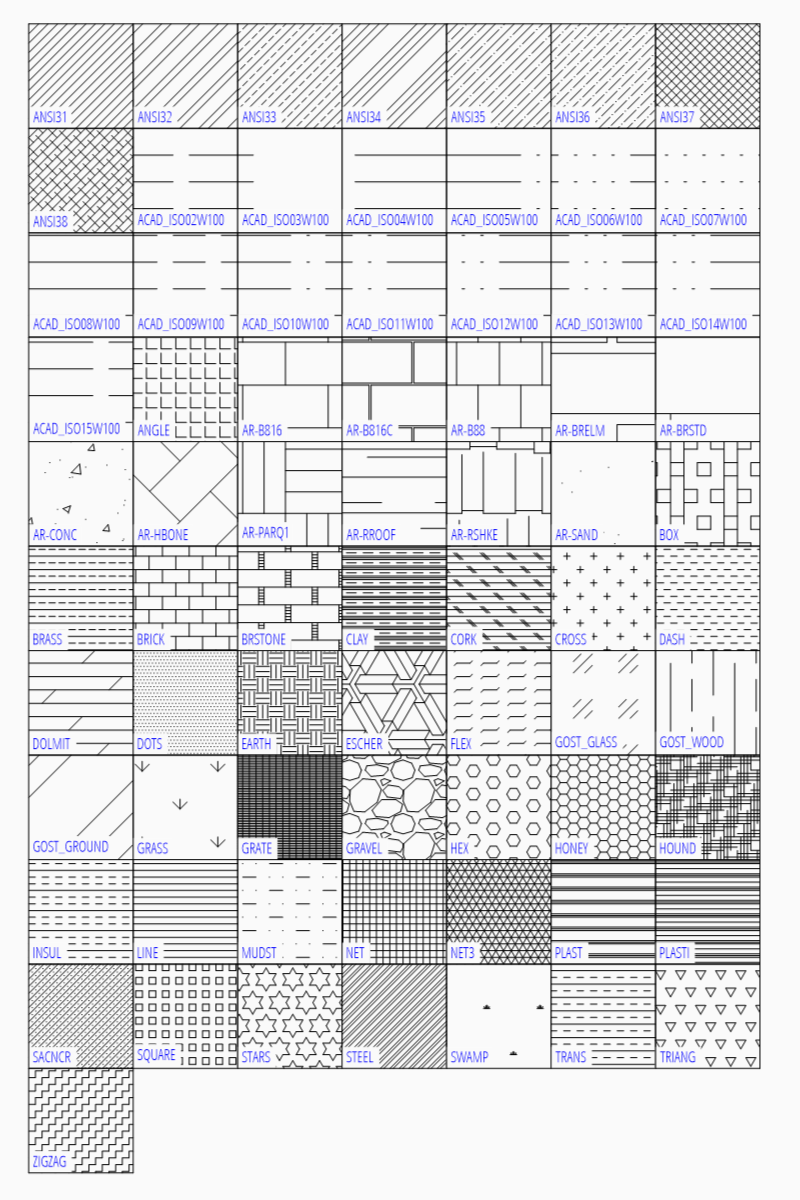TODO